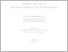Repository: Freie Universität Berlin, Math Department

# Gradient Methods for Membrane-Mediated Particle Interactions

Kies, Tobias (2018) Gradient Methods for Membrane-Mediated Particle Interactions. PhD Thesis . pp. 1-147.Preview

18MB

Official URL: https://refubium.fu-berlin.de/handle/fub188/24940

## Abstract

Outline In Chapter 1 the general model framework is introduced within which the di�erentiability is considered later on. In particular, also the interaction potential and other related quantities are de�ned there. This part is mainly based on the variational framework from [EGH+16, Hob16] but introduces a novel geometry-based formalism for the modeling of parametric particles, where the focus is on so-called curve and point value constraints. Concerning the elastic membrane energy, a Canham{Helfrich type functional is chosen as a root model, which subsequently is simpli�ed by parameterization and linearization techniques. Most importantly, in the absence of particles the membrane is generally assumed to be parameterized over a �xed reference domain. While the resulting framework is compatible with a wide array of possible non-linearized particle{ membrane interaction models, the main focus of attention lies on linearized models with either parametric curve or point value constraints during the remaining chapters. The core analytical results are shown in Chapter 2 where di�erentiability of the interaction potential for general parameterized energies is proven. Velocity methods from shape calculus are used in order to construct feasible domain perturbations that maintain the particle-induced constraints in an appropriate sense. The actual di�erentiability result is due to a transformation of the relevant function spaces and energies onto a reference set and an elementary application of the implicit function theorem. Under mild assumptions this even yields smoothness of the interaction potential in the interior of its domain of de�nition. Application of standard methods of matrix calculus enable the 10 derivation of a volume-representation of the gradient in dependence of the corresponding stationary membrane solution. The resulting analytic formula only contains terms that are both computable and controllable within a �nite element scheme, which makes it numerically feasible. Afterwards, Chapter 3 restricts the attention completely to linearized elliptic energies subject to curve or point value constraints. For the discrete approximation of stationary membranes subject to curve constraints a so-called �ctitious domain stabilized Nitsche method is introduced for shape-regular un�tted grids of quadrilaterals. For membranes subject to point value constraints a conforming �nite element discretization is introduced based on a standard Galerkin method with the help of local QR decompositions. In both cases optimal a priori error estimates are proven. In addition, it is also shown for such linearized models that the error in the discrete approximation of the interaction potential's gradient inherits the rate of convergence that is obtained for the discretization of the stationary membrane. Chapter 4 explains a possible implementation of these methods in conjunction with a level set ansatz for the description of parametric interfaces. It then proceeds to test previously obtained analytic error estimates for various di�erent examples. In particular, rates of convergence for the computation of stationary membrane shapes and for the approximation of the interaction potential gradient are explored and discussed with respect to their optimality and e�ciency. In Chapter 5 possible applications of the developed framework are demonstrated. The clustering behavior of isotropic and certain anisotropic particles is examined in Monge-gauge, and also the interaction of isotropic particles in a tubular membrane is illustrated. Moreover, a model for the membrane-mediated aggregation of FCHo2 FBAR domains is proposed based on physical data obtained from protein data base (PDB) �les. The computation of stationary con�gurations in those examples is accomplished via a perturbed gradient method. Finally, Chapter 6 gives a short outlook on a simple extension of the previously considered models to include thermal uctuations of particles via Langevin dynamics. This again leads naturally to the task of computing quantities from statistical mechanics such as free energies, which can be tackled with the help of now newly accessible gradienttype methods, such as the so-called energy perturbation method.

Item Type: Article Mathematical and Computer Sciences > Mathematics > Applied Mathematics Department of Mathematics and Computer Science > Institute of Mathematics 2472 Monika Drueck 19 Oct 2020 13:21 19 Oct 2020 13:21

Repository Staff Only: item control page